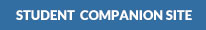Woodcock, Strachan:

Geological History of Britain and Ireland, 2nd Edition•Figures

•Tables (requires Adobe Acrobat Reader)

•Figures

•Figures

•Figures

•Figures

•Figures

•Figures

•Figures

•Figures

•Figures

•Figures

•Figures

•Figures

•Figures

•Figures

•Figures

•Figures

•Figures

•Figures

•Figures

•Figures

## Get Help With:

* These links will open a new window